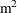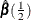Growth Charts for Body Mass Index

Body mass index (BMI) is defined as the ratio of weight (kg) to squared height (m) and is a widely used measure for categorizing individuals as overweight or underweight. The percentiles of BMI for specified ages are of particular interest. As age increases, these percentiles provide growth patterns of BMI not only for the majority of the population, but also for underweight or overweight extremes of the population. In addition, the percentiles of BMI for a specified age provide a reference for individuals at that age with respect to the population.

Smooth quantile curves have been widely used for reference charts in medical diagnosis to identify unusual subjects, whose measurements lie in the tails of the reference distribution. This example explains how to use the QUANTREG procedure to create growth charts for BMI.

A SAS data set named bmimen was created by merging and cleaning the 1999–2000 and 2001–2002 survey results for men published by the National Center for Health Statistics. This data set contains the variables Weight (kg), Height (m), BMI (kg/), Age (year), and SeQN (respondent sequence number) for 8,250 men. More details can be found in Chen (2005).

The data set used in this example is a subset of the original data set of Chen (2005). It contains the two variables BMI and Age with 3264 observations.

data bmimen;
input BMI Age @@;
SqrtAge = sqrt(Age);
InveAge = 1/Age;
LogBMI  = log(BMI);
datalines;
18.6  2.0 17.1  2.0 19.0  2.0 16.8  2.0 19.0  2.1  15.5   2.1
16.7  2.1 16.1  2.1 18.0  2.1 17.8  2.1 18.3  2.1  16.9   2.1
15.9  2.1 20.6  2.1 16.7  2.1 15.4  2.1 15.9  2.1  17.7   2.1

... more lines ...

29.0 80.0 24.1 80.0 26.6 80.0 24.2 80.0 22.7 80.0  28.4  80.0
26.3 80.0 25.6 80.0 24.8 80.0 28.6 80.0 25.7 80.0  25.8  80.0
22.5 80.0 25.1 80.0 27.0 80.0 27.9 80.0 28.5 80.0  21.7  80.0
33.5 80.0 26.1 80.0 28.4 80.0 22.7 80.0 28.0 80.0  42.7  80.0
;

The logarithm of BMI is used as the response (although this does not improve the quantile regression fit, it helps with statistical inference.) A preliminary median regression is fitted with a parametric model, which involves six powers of Age.

The following statements invoke the QUANTREG procedure:

proc quantreg data=bmimen algorithm=interior(tolerance=1e-5) ci=resampling;
model logbmi = inveage sqrtage age sqrtage*age
age*age age*age*age
/ diagnostics cutoff=4.5 quantile=.5 seed=1268;
id age bmi;
test_age_cubic: test age*age*age / wald lr rankscore(tau);
run;

The MODEL statement provides the model, and the option QUANTILE=0.5 requests median regression, which computesby using the interior point algorithm as requested with the ALGORITHM= option. See the section Interior Point Algorithm for details about this algorithm.

Figure 77.11 displays the estimated parameters, standard errors, 95% confidence intervals, t values, and p-values that are computed by the resampling method as requested by the CI= option. All of the parameters are considered significant since the p-values are smaller than 0.001.

Figure 77.11: Parameter Estimates with Median Regression: Men

The QUANTREG Procedure

Parameter Estimates
Parameter DF Estimate Standard Error 95% Confidence Limits t Value Pr > |t|
Intercept 1 7.8909 0.8168 6.2895 9.4924 9.66 <.0001
InveAge 1 -1.8354 0.4350 -2.6884 -0.9824 -4.22 <.0001
SqrtAge 1 -5.1247 0.7135 -6.5237 -3.7257 -7.18 <.0001
Age 1 1.9759 0.2537 1.4785 2.4733 7.79 <.0001
SqrtAge*Age 1 -0.3347 0.0424 -0.4179 -0.2515 -7.89 <.0001
Age*Age 1 0.0227 0.0029 0.0170 0.0284 7.77 <.0001
Age*Age*Age 1 -0.0000 0.0000 -0.0001 -0.0000 -7.40 <.0001

The TEST statement requests Wald, likelihood ratio, and rank tests for the significance of the cubic term in Age. The test results, shown in Figure 77.12, indicate that this term is significant. Higher-order terms are not significant.

Figure 77.12: Test of Significance for Cubic Term

Test test_age_cubic Results
Test Test Statistic DF Chi-Square Pr > ChiSq
Wald 54.7417 1 54.74 <.0001
Likelihood Ratio 56.9473 1 56.95 <.0001
Rank_Tau 42.5731 1 42.57 <.0001

Median regression and, more generally, quantile regression are robust to extremes of the response variable. The DIAGNOSTICS option in the MODEL statement requests a diagnostic table of outliers, shown in Figure 77.13, which uses a cutoff value specified with the CUTOFF= option. The variables specified in the ID statement are included in the table.

With CUTOFF=4.5, 14 men are identified as outliers. All of these men have large positive standardized residuals, which indicates that they are overweight for their age. The cutoff value 4.5 is ad hoc; it corresponds to a probability less than 0.5E–5 if normality is assumed, but the standardized residuals for median regression usually do not meet this assumption.

In order to construct the chart shown in Figure 77.2, the same model used for median regression is used for other quantiles. Note that the QUANTREG procedure can compute fitted values for multiple quantiles.

Figure 77.13: Diagnostics with Median Regression

Diagnostics
Obs Age BMI Standardized
Residual
Outlier
1337 8.900000 36.500000 5.3575 *
1376 9.200000 39.600000 5.8723 *
1428 9.400000 36.900000 5.3036 *
1505 9.900000 35.500000 4.8862 *
1764 14.900000 46.800000 5.6403 *
1838 16.200000 50.400000 5.9138 *
1845 16.300000 42.600000 4.6683 *
1870 16.700000 42.600000 4.5930 *
1957 18.100000 49.900000 5.5053 *
2002 18.700000 52.700000 5.8106 *
2016 18.900000 48.400000 5.1603 *
2264 32.000000 55.600000 5.3085 *
2291 35.000000 60.900000 5.9406 *
2732 66.000000 14.900000 -4.7849 *

The following statements request fitted values for 10 quantile levels ranging from 0.03 to 0.97:

proc quantreg data=bmimen algorithm=interior(tolerance=1e-5) ci=none;
model logbmi = inveage sqrtage age sqrtage*age
age*age age*age*age
/ quantile=0.03,0.05,0.1,0.25,0.5,0.75,
0.85,0.90,0.95,0.97;
output out=outp pred=p/columnwise;
run;

data outbmi;
set outp;
pbmi = exp(p);
run;

proc sgplot data=outbmi;
title 'BMI Percentiles for Men: 2-80 Years Old';
yaxis label='BMI (kg/m**2)' min=10 max=45 values=(10 15 20 25 30 35 40 45);
xaxis label='Age (Years)' min=2 max=80 values=(2 10 20 30 40 50 60 70 80);

scatter x=age y=bmi /markerattrs=(size=1);
series  x=age y=pbmi/group=QUANTILE;
run;

The fitted values are stored in the OUTPUT data set outp. The COLUMNWISE option arranges these fitted values for all quantiles in the single variable p by groups of the quantiles. After the exponential transformation, the fitted BMI values together with the original BMI values are plotted against age to create the display shown in Figure 77.2.

The fitted quantile curves reveal important information. During the quick growth period (ages 2 to 20), the dispersion of BMI increases dramatically; it becomes stable during middle age, and then it contracts after age 60. This pattern suggests that effective population weight control should start in childhood.

Compared to the 97th percentile in reference growth charts published by CDC in 2000 (Kuczmarski, Ogden, and Guo, 2002), the 97th percentile for 10-year-old boys in Figure 77.2 is 6.4 BMI units higher (an increase of 27%). This can be interpreted as a warning of overweight or obesity. See Chen (2005) for a detailed analysis.